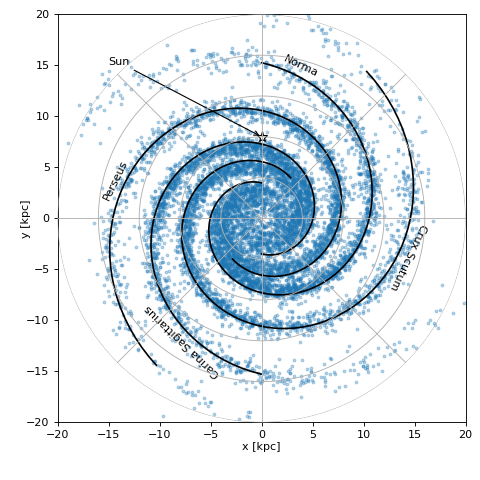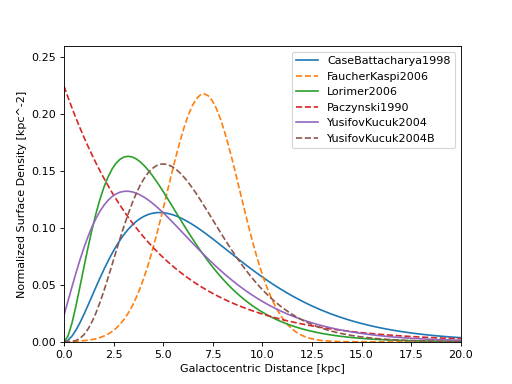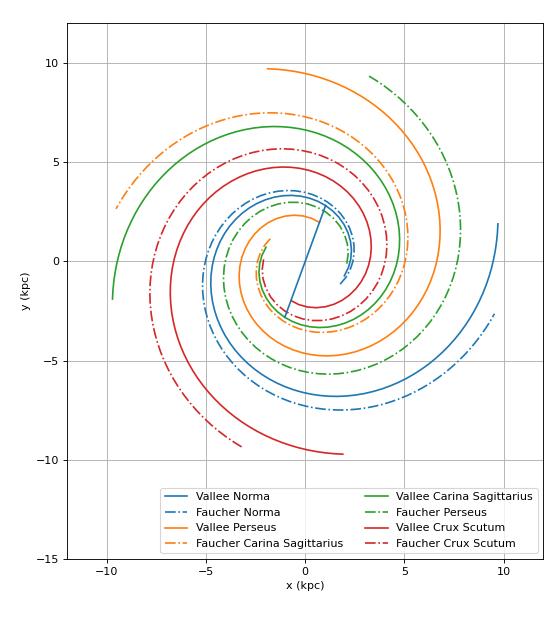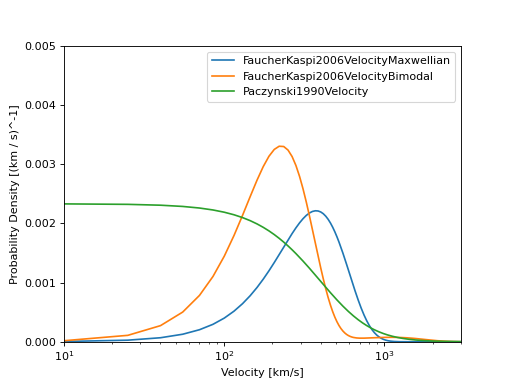# Astrophysical source population models (gammapy.astro.population)¶

## Introduction¶

The gammapy.astro.population module provides a simple framework for population synthesis of gamma-ray sources, which is useful in the context of surveys and population studies.

## Getting Started¶

The following example illustrates how to simulate a basic catalog including a spiral arm model.

import astropy.units as u
from gammapy.astro.population import make_base_catalog_galactic

max_age = 1E6 * u.yr
SN_rate = 3. / (100. * u.yr)
n_sources = int(max_age * SN_rate)
table = make_base_catalog_galactic(
n_sources=n_sources,
vel_dis='F06B',
max_age=max_age,
spiralarms=True,
)


The total number of sources is determined assuming a maximum age and a supernova rate. The table returned is an instance of Table which can be used for further processing. The example population with spiral-arms is illustrated in the following plot.

(png, hires.png, pdf)### Galactocentric spatial distributions¶

Here is a comparison plot of all available radial distribution functions of the surface density of pulsars and related objects used in literature:

(png, hires.png, pdf)TODO: add illustration of Galactocentric z-distribution model and combined (r, z) distribution for the Besancon model.

### Spiral arm models¶

Two spiral arm models of the Milky way are available: ValleeSpiral and gammapy.astro.population.FaucherSpiral

(png, hires.png, pdf)### Velocity distributions¶

Here is a comparison plot of all available velocity distribution functions:

(png, hires.png, pdf)## Reference/API¶

### gammapy.astro.population Package¶

Astrophysical population models.

#### Functions¶

 add_observed_parameters(table[, obs_pos]) Add observable parameters (such as sky position or distance). add_pulsar_parameters(table[, B_mean, …]) Add pulsar parameters to the table. add_pwn_parameters(table) Add PWN parameters to the table. add_snr_parameters(table) Add SNR parameters to the table. make_base_catalog_galactic(n_sources[, …]) Make a catalog of Galactic sources, with basic source parameters. make_catalog_random_positions_cube([size, …]) Make a catalog of sources randomly distributed on a line, square or cube. Sample random source locations in a sphere.

#### Classes¶

 CaseBattacharya1998([amplitude, alpha, beta]) Radial distribution of the surface density of supernova remnants in the galaxy - Case & Battacharya 1998. Exponential([amplitude, z_0]) Exponential distribution. FaucherKaspi2006([amplitude, r_0, sigma]) Radial distribution of the birth surface density of pulsars in the galaxy - Faucher-Giguere & Kaspi 2006. FaucherKaspi2006VelocityBimodal([amplitude, …]) Bimodal pulsar velocity distribution - Faucher & Kaspi (2006). Maxwellian pulsar velocity distribution. FaucherSpiral Milky way spiral arm used in Faucher et al (2006). LogSpiral Logarithmic spiral. Lorimer2006([amplitude, B, C]) Radial distribution of the surface density of pulsars in the galaxy - Lorimer 2006. Paczynski1990([amplitude, r_exp]) Radial distribution of the birth surface density of neutron stars - Paczynski 1990. Paczynski1990Velocity([amplitude, v_0]) Distribution by Lyne 1982 and adopted by Paczynski and Faucher. Milky way spiral arm model from Vallee (2008). YusifovKucuk2004([amplitude, a, b, r_1]) Radial distribution of the surface density of pulsars in the galaxy - Yusifov & Kucuk 2004. YusifovKucuk2004B([amplitude, a, b]) Radial distribution of the surface density of OB stars in the galaxy - Yusifov & Kucuk 2004.